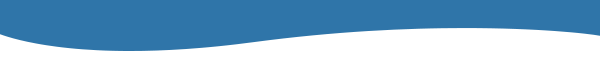# What Is Quantum Mechanics Essay

explanatory Essay
887 words
887 wordsCherop Soi
Mrs. Foley
Physics
November 14, 2017 Quantum Mechanics

Quantum Mechanics developed over many decades beginning as a set of controversial mathematical explanations of experiments that the math of classical mechanics could not explain. It began in the turn of the 20th century, a separate mathematical revolution in physics that describes the motion of things at high speeds. The origins of Quantum Mechanics cannot be credited to any one scientists. Multiple scientists contributed to a foundation of three revolutionary principles that gradually gained acceptance and experiment verification from 1900-1930 (Coolman). Quantum Mechanics is

Quantum Mechanics is a branch of physics that describes the structure and behavior of matter. …show more content…

#### In this essay, the author

• Explains that quantum mechanics developed over many decades beginning as a set of controversial mathematical explanations of experiments that the math of classical mechanics could not explain.
• Explains quantum mechanics is a branch of physics that describes the structure and behavior of matter. it replaced classical mechanics, based on physical laws described by sir isaac newton and james clerk maxwell.
• Explains that quantum mechanics describes objects and phenomena that seem strange and are difficult to understand, such as quantum energy, wave-particle duality, and the uncertainty principle.
• Explains how max planck found the first flaw in classical mechanics. scientists measured the amounts of energy in different wavelengths of light emitted from the surfaces of certain hot objects.
• Explains that certain properties, such as position, speed and color, can sometimes only occur in specific, set amounts. this challenged the assumption of classical mechanics, which said such properties should exist on a smooth, continuous spectrum.
• Describes planck's equation to explain the distribution of colors emitted over the spectrum in the glow of red and white-hot objects, which was unexpected because light was understood to act as a wave.
• Explains how quantization helped explain other mysteries of physics. in 1907, einstein used planck's hypothesis of quantization to explain why the temperature changed by different amounts if you put the same amount of heat into the material.
• Explains that light behaves as a wave, like ripples on the surface of calm lakes. added wave crests result in brighter light, while waves that cancel out produce darkness.
• Explains that in 1924, louis de broglie proposed that subatomic particles, such as electrons, are associated with waves. later experiments determined him correct.

Quantum mechanics describes several objects and phenomena that seem strange and are difficult to understand. Among these are quanta “chunks” of energy; the wave-particle duality of matter; and the uncertainty principle which limits what we can know about objects. In 1927, German physicist Werner Heisenberg discovered a general characteristic of quantum mechanics, the uncertainty principle. According to this principle, it is impossible to precisely describe both the location and the momentum of a particle at the same time, therefore it affects the attempts to measure a particle’s location and …show more content…

In 1907, Einstein used Planck’s hypothesis of quantization to explain why the temperature of a solid changed by different amounts if you put the same amount of heat into the material. Since the early 1800’s, the science of spectroscopy had shown that different elements emit and absorb specific colors of light called “spectral lines.” In 1888, Johannes Rydberg derived an equation that described the spectral lines emitted by hydrogen, though nobody could explain why the equation worked. This changed in 1913 when Danish physicist Niel Bohr applied Planck’s hypothesis of quantization to Ernest Rutherford’s 1911 “planetary” model of the atom, which affirmed that electrons orbited the nucleus the same way that planets orbit the sun. Bohr offered an explanation for why electrical attraction does not make the electrons spiral into the nucleus. He said that electrons in atoms can change their energy only by absorbing or emitting quanta. When an electron absorbs a quantum it moves quickly to orbit farther from nucleus. When an electron emits a quantum the electron jumps to a closer